# Sugar production¶

The problem description in this section is taken from Section 6.4 Cane sugar production of the book Applications of optimization with Xpress-MP. The harvest of cane sugar in Australia is highly mechanized. The sugar cane is immediately transported to a sugarhouse in wagons that run on a network of small rail tracks. The sugar content of a wagonload depends on the field it has been harvested from and on the maturity of the sugar cane. Once harvested, the sugar content decreases rapidly through fermentation and the wagonload will entirely lose its value after a certain time. At this moment, eleven wagons loaded with the same quantity have arrived at the sugarhouse. They have been examined to find out the hourly loss and the remaining life span (in hours) of every wagon, these data are summarized in the following table:

 Lot 1 2 3 4 5 6 7 8 9 10 11 Loss (kg/h) 43 26 37 28 13 54 62 49 19 28 30 Life span (h) 8 8 2 8 4 8 8 8 8 8 8

Every lot may be processed by any of the three, fully equivalent production lines of the sugarhouse. The processing of a lot takes two hours. It must be finished at the latest at the end of the life span of the wagonload. The manager of the sugarhouse wishes to determine a production schedule for the currently available lots that minimizes the total loss of sugar.

## Model formulation¶

Let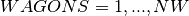be the set of wagons,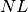the number of production lines and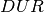the duration of the production process for every lot. The hourly loss for every wagon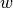is given by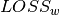and its life span by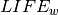. We observe that, in an optimal solution, the production lines need to work without any break otherwise we could reduce the loss in sugar by advancing the start of the lot that follows the break. This means that the completion time of every lot is of the form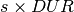, with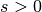and is an integer. The maximum value of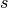is the number of time slots (of length) that the sugarhouse will work, namely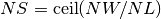, where ceil stands for rounded to the next largest integer. If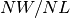is an integer, every line will process exactly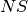lots. Otherwise, some lines will process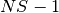lots, but at least one line processeslots. In all cases, the length of the optimal schedule is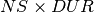hours. We call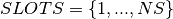the set of time slots. Every lot needs to be assigned to a time slot. We define variables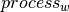for the time slot assigned to wagonand variables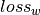for the loss incurred by this wagonload. Every time slot may take up tolots because there areparallel lines; therefore, we limit the number of occurrences of time slot values among thevariables (this constraint relation is often called cardinality constraint) :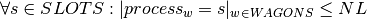The loss of sugar per wagonloadand time slotis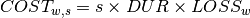. Let variablesdenote the loss incurred by wagon load: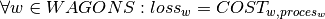The objective function (total loss of sugar) is then given as the sum of all losses: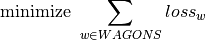## Implementation¶

The following model is the implementation of this problem. It uses the function ceil to calculate the maximum number of time slots. The constraints on the processing variables are expressed by occurrence relations and the losses are obtained via element constraints. The branching strategy uses the variable selection criterion KLargestMin, that is, choosing the variable with the largest lower bound.

// Number of wagon loads of sugar
int NW = 11;
// Number of production lines
int NL = 3;
// Time slots for production
int NS = ceil(NW/((float)NL));
// Loss in kg/hour
int LOSS[] = { 43 ,26, 37, 28, 13, 54, 62, 49, 19, 28 ,30};
// Remaining time per lot (in hours)
int LIFE[] =  { 8 , 8,  2,  8 , 4 , 8 , 8,  8,  6,  8 , 8};
// Cost per wagon
KIntArray COST;
// Duration of the production
int DUR = 2;

// Loss per wagon
KIntVarArray loss;

// Time slots for wagon loads
KIntVarArray process;

// Objective variable
KIntVar * totalLoss;

// Creation of the problem in this session
KProblem problem(session,"A-4 Cane sugar production 1");

int w;
// Variables creation
char name;
for (w=0;w<NW;w++) {
sprintf(name,"process(%i)",w);
process += (* new KIntVar(problem,name,0,NS-1) ) ;
}
for (w=0;w<NW;w++) {
sprintf(name,"loss(%i)",w);
loss += (* new KIntVar(problem,name,0,10000) ) ;
}

int s;
// Wagon loads per time slot
for (s=0; s < NS; s++) {
KOccurTerm oc(s, process);
problem.post(new KOccurrence(oc, NL, false, true));
}

// Limit on raw product life
for (w = 0; w < NW; w++) {
process[w].setSup(floor(LIFE[w]/((float)DUR))-1);
}

// initialization of COST array
for (s = 0; s < NS; s++) {
COST += 0;
}

KLinTerm totLossTerm;
// Objective function: total loss
for (w = 0; w < NW; w++) {
for (s=0;s<NS;s++) {
COST[s] = (s+1)*DUR*LOSS[w];
}
KEltTerm kelt(COST,process[w]);
problem.post(new KElement(kelt, loss[w],"element"));
totLossTerm = totLossTerm + loss[w];
}
totalLoss = new KIntVar(problem,"totalLoss",0,10000);
problem.post(totLossTerm == *totalLoss);

// propagating problem
if (problem.propagate()) {
printf("Problem is infeasible\n");
exit(1);
}

// search strategy customization
KBranchingSchemeArray myBa;
myBa += KAssignVar(KSmallestMax(),KMinToMax(),process);

// Solve the problem
// creation of the solver
KSolver solver(problem,myBa);

// setting objective and sense of optimization
problem.setSense(KProblem::Minimize);
problem.setObjective(*totalLoss);

int result = solver.optimize();
// solution printing
KSolution * sol = &problem.getSolution();
// print solution resume
sol->printResume();

// Solution printing
printf("Total loss: %i\n", sol->getValue(*totalLoss));
for (s = 0; s < NS; s++) {
printf("Slot %i: ", s);
for (w=0;w<NW;w++) {
if (sol->getValue(process[w]) == s) {
printf("wagon %i (%i) ",w,(s+1)*DUR*LOSS[w]);
}
}
printf("\n");
}


An alternative formulation of the constraints on the processing variables is to replace the KOccurrence constraints by a single KGlobalCardinalityConstraint, indicating for every time slot the minimum and maximum number (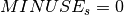and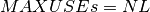) of production lines that may be used.

int * vals = new int[NS];
int * low = new int[NS];
int * upp = new int[NS];
for (s=0;s<NS;s++) {
vals[s] = s;
low[s] = 0;
upp[s] = NL;
}
problem.post(new KGlobalCardinalityConstraint("Wagon_loads_per_time_slot\0", process, vals, low, upp,  NS));
delete[] vals;
delete[] low;
delete[] upp;


Yet another formulation of this problem is possible with Artelys Kalis, namely interpreting it as a cumulative scheduling problem where the wagon loads are represented by tasks of unit duration, scheduled on a discrete resource with a capacity corresponding to the number of production lines.

## Results¶

We obtain a total loss of 1620 kg of sugar. The corresponding schedule of lots is shown in the following table (there are several equivalent solutions) :

 Slot 1 Slot 2 Slot 3 Slot 4 lot 3 (74 kg) lot 1 (172 kg) lot 4 (168 kg) lot 2 (208 kg) lot 6 (108 kg) lot 5 (52 kg) lot 9 (114 kg) lot 10 (224 kg) lot 7 (124 kg) lot 8 (196 kg) lot 11 (180 kg)